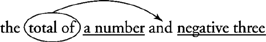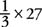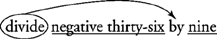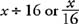## Keywords for Mathematical Operations

The first step in solving a word problem is always to read the problem. You need to be able to translate words into mathematical symbols, focusing on keywords that indicate the mathematical procedures required to solve the problem—both the operation and the order of the expression. In much the same way that you can translate Spanish into English, you can translate English words into symbols, the language of mathematics. Many (if not all) keywords that indicate mathematical operations are familiar words.

To begin, you translate English phrases into algebraic expressions. An algebraic expression is a collection of numbers, variables, operations, and grouping symbols. You will translate an unknown number as the variable x or n. The grouping symbols are usually a set of parentheses, but they can also be sets of brackets or braces.

In translating expressions, you want to be well acquainted with basic keywords that translate into mathematical operations: addition keywords, subtraction keywords, multiplication keywords, and division keywords, which are covered in the four following sections.

Some common examples of addition keywords are as follows:

• SUM OF_____ AND _____
• TOTAL OF _____ AND _____
• _____ PLUS _____
• _____ INCREASED BY _____
• GAIN
• RAISE
• MORE
• INCREASE OF

The first two keywords (SUM and TOTAL) are called leading keywords because they lead the expression. The second two keywords (PLUS and INCREASED BY) are keywords that indicate the exact placement of the plus sign. The last four keywords can be found in word problems and may indicate addition.

When an expression begins with the leading keywords SUM or TOTAL, the leading keyword defines the corresponding AND. The plus sign then physically replaces the AND in the expression.

Example 1: Translate the following: the sum of five and a number

1. Underline the words before and after AND when it corresponds to the leading keyword SUM OF.

• the sum of five and a number

2. Circle the leading keyword and indicate the corresponding AND that it defines.3. Translate each underlined expression and replace AND with a plus sign.

• The expression translates to 5 + x.

Example 2: Translate the following: the total of a number and negative three

Use the following steps to translate this problem:

1. The keyword TOTAL OF is a leading keyword that defines AND, so underline the words before and after AND: “a number” and “negative three.”

• the total of a number and negative three

2. Circle the leading keyword and indicate the corresponding AND that it defines.3. Translate each underlined expression and replace AND with a plus sign.

• The expression translates to x + −3.

Example 3: Translate the following: the sum of seven and negative four

Translate this example in the following way:

1. The word SUM OF is a leading keyword that defines AND, so underline the words before and after AND: “seven” and “negative four.”

• the sum of seven and negative four

2. Circle the leading keyword and indicate the corresponding AND that it defines.3. Translate each underlined expression and replace AND with a plus sign.

• The expression translates to 7 + −4.

Reminder: The AND keyword translates to mean “plus” because the leading keyword is SUM OF. With other leading keywords (discussed in the following sections), AND can mean other things. Also notice that you do not simplify the expression and get “3” for the answer because you are just translating words into symbols and not performing the math.

Two other keywords on the addition keyword list, PLUS and INCREASED BY, can be correctly translated by the direct translation strategy. In the direct translation strategy, you translate each word into its corresponding algebraic symbol, one at a time, in the same order as written, as shown in Example 4.

Example 4: Translate the following: a number increased by twenty‐four

• The expression translates to x + 24.

Some additional keywords, such as GAIN, MORE, INCREASE OF, and RAISE, are commonly found in story problems, as in Example 5.

Example 5: Translate the following story problem into a mathematical expression about the weight of the linebacker: The defensive linebacker weighed two hundred twenty‐two pounds at the beginning of spring training. He had a gain of seventeen pounds after working out with the team for four weeks.

• The expression translates to 222 + 17.

Note: Not all numbers mentioned in a word problem should be included in the mathematical expression. The number “four” is just interesting fact, but it is not information you need in order to write an expression about the linebacker's weight.

You may also be wondering why the answer isn't 239 pounds. That's because the question asks you to translate the story problem into a mathematical expression, not to evaluate the expression.

Example 6: Translate the following word problem into a mathematical expression about the cashier's current hourly wage: A cashier at the corner grocery was earning \$6.25 an hour. He received a raise of 25 cents an hour.

• The expression translates to 6.25 + 0.25.

Note: The hourly wage is stated in dollars, and the raise is stated in cents. Any time you are adding two numbers that have units, make sure both numbers are measured with the same units; if they aren't, convert one of the numbers to the same units as the other. Having both numbers measured with the same units is called homogeneous units. In this example, you convert his raise, the 25 cents, to \$0.25 because his hourly wage is measured in dollars, not cents, so the raise must also be in dollars.

## Subtraction keywords

Subtraction keywords also include leading keywords, keywords that can be translated one word at a time, and keywords that are found in story problems. Look at the following list of subtraction keywords:

• DIFFERENCE BETWEEN _____ AND _____
• _____ MINUS _____
• _____ DECREASED BY _____
• LOSS
• LESS
• FEWER
• TAKE AWAY

One subtraction keyword (DIFFERENCE BETWEEN) is a two‐part expression that begins with a leading keyword that defines the corresponding AND. You can use the same methods of underlining and circling the keywords shown in the preceding section to translate these expressions.

Example 7: Translate the following: the difference between four and six

Here is how you translate Example 7:

1. Because the keyword DIFFERENCE BETWEEN is a leading keyword that defines the corresponding AND, underline the words before and after AND: “four” and “six.”

• the difference between four and six

2. Circle the leading keyword and indicate the corresponding AND that it defines.3. Translate each underlined expression and replace AND with a minus sign.

• The expression translates to 4 – 6.

Note: AND is not always translated to mean addition. Here, the DIFFERENCE BETWEEN is the leading keyword that defines the AND to mean subtraction.

Other subtraction keywords, such as MINUS and DECREASED BY, use the direct translation strategy. Example 8 is a subtraction word problem that is translated one keyword at a time, in the exact order of the expression.

Example 8: Translate the following: twenty‐four decreased by a number

• The expression translates to 24 – x.

In a story problem, you may find the subtraction keywords LOSS, LESS, FEWER, and TAKE AWAY, as shown in Example 9.

Example 9: Translate the following word problem into a mathematical expression about the current value of materials at the job site: A construction company stored \$1,253 worth of materials at the job site. The company suffered a loss of \$300 due to storm damage.

• The expression translates to 1,253 – 300.

## Multiplication keywords

Some common examples of multiplication keywords are as follows:

• MULTIPLY _____ BY _____
• PRODUCT OF _____ AND _____
• _____ TIMES _____
• DOUBLE _____
• TWICE _____
• TRIPLE _____
• PERCENT OF _____
• FRACTION OF _____

For two of the multiplication keywords, MULTIPLY and PRODUCT OF, a leading keyword defines the corresponding BY or AND, as shown in Example 10.

Example 10: Translate the following: the product of seven and a number

Translate this example in the following way:

1. Because PRODUCT OF is a leading keyword that corresponds to AND, underline the words before and after AND: “seven” and “a number.”

• the product of seven and a number

2. Circle the leading keyword and indicate the corresponding AND that it defines.3. Translate each underlined expression and replace AND with a times sign.

• The expression translates to 7 × x.

Note: Keep in mind that AND does not always indicate addition. The keyword PRODUCT OF defines the AND in this expression to mean multiplication.

A multiplication expression that is translated by the direct translation method is shown in Example 11.

Example 11: Translate the following: a number times fifteen

The expression translates to x × 15.

Some multiplication keywords, such as DOUBLE, TWICE, and TRIPLE, translate into a number and the operation of multiplication, as shown in Examples 12 and 13.

Example 12: Translate the following: twice a number

The expression translates to 2 × x.

Example 13: Translate the following word problem into a mathematical expression: Jennifer had \$15 dollars in the bank. Over the next two weeks she doubled her money.

The expression translates to 2 × 15.

One of the keywords that indicates multiplication is OF. In word problems, however, you may see more than one use of the word “of.” The only OF that indicates multiplication is the one that follows the keyword PERCENT, the percent sign, the keyword FRACTION, or a fraction. See Examples 14 and 15.

Example 14: Translate the following: twenty five percent of four hundred dollars

The expression translates to 0.25 × 400.

Note: Remember that a percent is changed to a decimal before multiplying.

Example 15: Translate the following: one‐third of twenty‐seven

The expression translates to.

## Division keywords

Some common examples of division keywords are as follows:

• QUOTIENT OF _____ AND _____
• DIVIDE _____ BY _____
• _____ DIVIDED BY _____
• DIVIDED EQUALLY
• PER

The keywords PRODUCT OF and QUOTIENT OF are difficult for some people to differentiate. Here is a hint to help you remember which one indicates division and which one indicates multiplication: QUOTIENT is a “harder” word than “PRODUCT,” and division is a “harder” operation than multiplication.

Remember: Leading keywords define the corresponding AND or BY to mean divide, usually designated with the symbol ÷.

Example 16: Translate the following: the quotient of seven and a number

1. Because the keyword QUOTIENT OF is a leading keyword that defines AND, underline the words before and after AND: “seven” and “a number.”

• the quotient of seven and a number

2. Circle the leading keyword and indicate the corresponding AND that it defines.3. Translate each underlined expression and replace AND with a division sign.

• The expression translates to 7 ÷ n.

Note: Here, the keyword QUOTIENT OF defines AND to mean division.

Example 17: Translate the following: divide negative thirty‐six by nine

1. Because the word DIVIDE is a leading keyword that defines the BY, underline the words before and after BY: “negative thirty‐six” and “nine.”

• divide negative thirty‐six by nine

2. Circle the leading keyword and indicate the corresponding BY that it defines.3. Translate each underlined expression and replace BY with a division sign.

• The expression translates to.

Note: The first number goes in the numerator when using a fraction bar to indicate division. The number in the numerator (the −36) goes inside the “house” when using the long division symbol.

Some division keywords can be translated one word at a time. Instead, you just follow the sentence and replace with algebraic notations as you go along.

Example 18: Translate the following: a number divided by 16

The expression translates to.

Often, in story problems, the keyword that indicates division is PER. When a story problem asks for the speed of a vehicle in miles per hour, set up the expression to divide the number of miles by the number of hours. You not only directly translate “miles” ÷ “hours,” but also identify the number of miles and number of hours by finding them elsewhere in the problem. See Example 19.

Example 19: Translate the following word problem into a mathematical expression about speed: It takes three hours to travel 150 miles to grandmother's house. How do you find your average speed in miles per hour?

You find “miles” ÷ “hours” in the question. In the first part of the word problem, you find the number of miles, 150 miles, and the number of hours, three hours.

The expression translates to 150 ÷ 3.